Next: Dot product test Up: Adjoint operators Previous: Causal integration

Consider a modeland an operatorwhich creates some theoretical data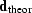.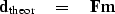(13)
The general task of geophysicists is to begin from observed dataand find an estimated model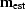that satisfies the simultaneous equations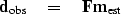(14)
This is the topic of a large discipline variously called inversion'' or estimation''. Basically, it defines a residual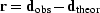and then minimizes its length.Findingthis way is called the least squares method. The basic result (not proven here) is that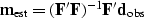(15)
In many cases including all seismic imaging cases, the matrixis far too large to be invertible. People generally proceed by a rough guess at an approximation for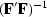.The usual first approximation is the optimistic one that.To this happy approximation, the inverse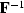is the adjoint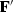.

In this book we'll see examples where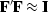is a good approximation and other examples where it isn't. We can tell how good the approximation is. We take some hypothetical data and convert it to a model, and use that model to make some reconstructed data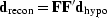.Likewise we could go from a hypothetical model to some data and then to a reconstructed model.Luckily, it often happens that the reconstructed differs from the hypothetical in some trivial way, like by a scaling factor, or by a scaling factor that is a function of physical location or time, or a scaling factor that is a function of frequency. It isn't always simply a matter of a scaling factor, but it often is, and when it is, we often simply redefine the operator to include the scaling factor. Observe that there are two places for scaling functions (or filters), one in model space, the other in data space.

We could do better than the adjoint by iterative modeling methods (conjugate gradients) that are also described elsewhere. These methods generally demand that the adjoint be computed correctly. As a result, we'll be a little careful about adjoints in this book to compute them correctly even though this book does not require them to be exactly correct.Next: Dot product test Up: Adjoint operators Previous: Causal integration
Stanford Exploration Project
12/26/2000